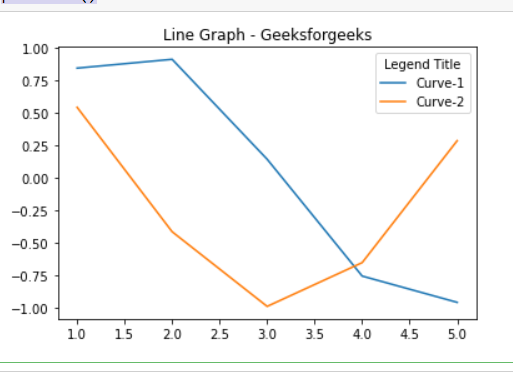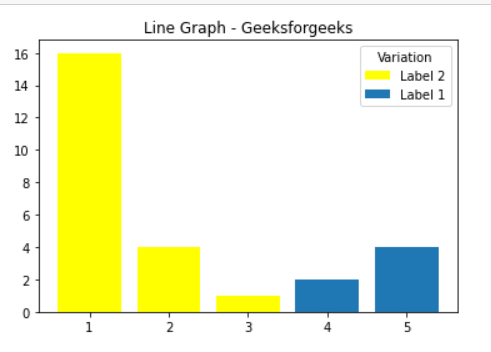Related Articles
How to add a title to a Matplotlib legend?
• Last Updated : 24 Jan, 2021

Prerequisites: Matplotlib

In this article, we will see how can we can add a title to a legend in our graph using matplotlib, Here we will take two different examples to showcase our graph.

Approach:

• Import required module.
• Create data.
• Add a title to a legend.
• Normally plot the data.
• Display plot.

Below is the Implementation:

Example 1:

In this example, we will draw different lines with the help of matplotlib and Use the title argument to plt.legend() to specify the legend title.

## Python3

 `# importing package``import` `matplotlib.pyplot as plt``import` `numpy as np`` ` `# create data``X ``=` `[``1``, ``2``, ``3``, ``4``, ``5``]`` ` `# plot lines``plt.plot(X, np.sin(X), label ``=` `"Curve-1"``)``plt.plot(X, np.cos(X), label ``=` `"Curve-2"``)`` ` `# Add a title to a legend``plt.legend(title ``=` `"Legend Title"``)``plt.title(``"Line Graph - Geeksforgeeks"``)`` ` `plt.show()`

Output:Example 2:

In this example, we will draw a Bar Graph with the help of matplotlib and Use the title argument to plt.legend() to specify the legend title.

## Python3

 `# importing package``import` `matplotlib.pyplot as plt`` ` `# sample code ``plt.bar([``1``, ``2``, ``3``], [``16``, ``4``, ``1``], ``        ``color ``=``'yellow'``,``        ``label ``=` `'Label 2'``) `` ` `plt.bar([``4``, ``5``], [``2``, ``4``], ``        ``label ``=` `'Label 1'``)`` ` `# Add a title to a legend``plt.legend(title ``=` `"Variation Rate"``)`` ` `plt.title(``"Line Graph - Geeksforgeeks"``)`` ` `plt.show()`

Output:Attention geek! Strengthen your foundations with the Python Programming Foundation Course and learn the basics.

To begin with, your interview preparations Enhance your Data Structures concepts with the Python DS Course. And to begin with your Machine Learning Journey, join the Machine Learning – Basic Level Course

My Personal Notes arrow_drop_up